Practice the problems of Math in Focus Grade 3 Workbook Answer Key Chapter 10 Practice 2 Addition to score better marks in the exam.

Complete each number bond. Then add.

Example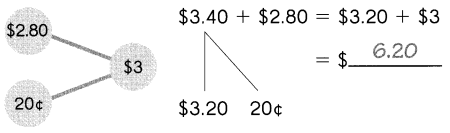Question 1.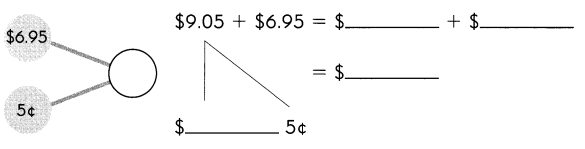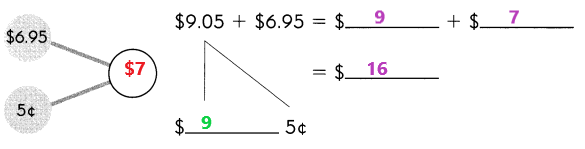Explanation:
To make $6.95 to the nearest integer we have to add required ¢ to the$6.95.
A number bond is a simple addition of two numbers that add up to give the sum. Add these two numbers $6.95 and 5¢ the sum is$7.
The number $9.05 is divided into two parts as$9 and 5¢.
In place of $9.05 and$6.95 we have to use $9 and$7.
Add $9 with$7 the sum is $16. Question 2.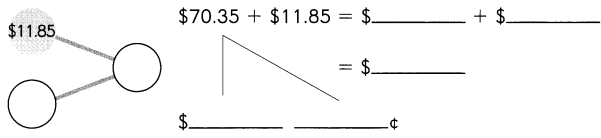Answer: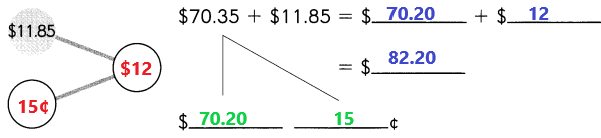Explanation: To make$11.85 to the nearest integer we have to add required ¢ to the $11.85. A number bond is a simple addition of two numbers that add up to give the sum. Add these two numbers$11.85 and 15¢ the sum is $12. The number$70.35 is divided into two parts as $70.20 and 15¢. In place of$70.35 and $11.85 we have to use$70.20 and $12. Add$70.20 with $12 the sum is$82.20.

Question 3.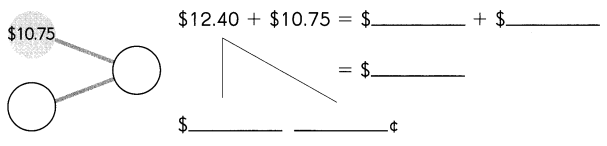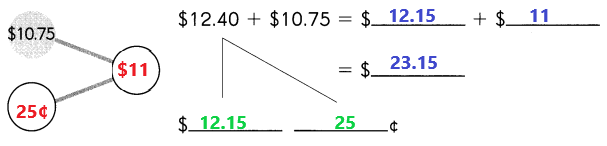Explanation:
To make $10.75 to the nearest integer we have to add required ¢ to the$10.75.
A number bond is a simple addition of two numbers that add up to give the sum. Add these two numbers $10.75 and 25¢ the sum is$11.
The number $12.40 is divided into two parts as$12.150 and 25¢.
In place of $12.40 and$10.75 we have to use $12.15 and$11.
Add $12.15 with$11 the sum is $23.15. Complete each number bond. Then add. Example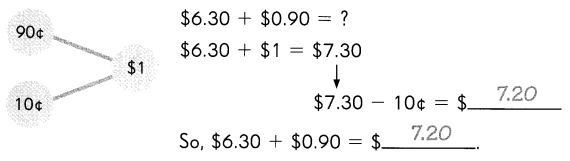Question 4.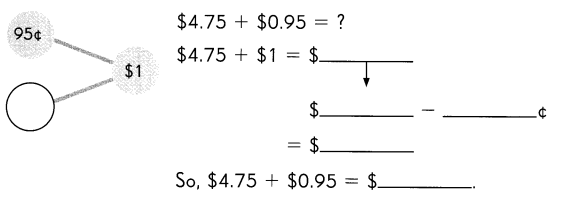Answer: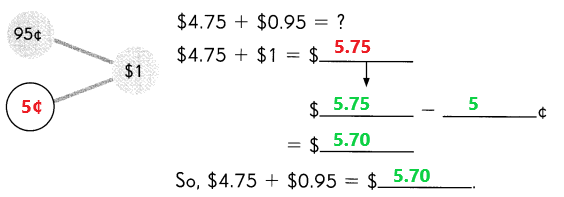Explanation: To make$0.95 to the nearest integer we have to add required ¢ to the $0.95. A number bond is a simple addition of two numbers that add up to give the sum. Add these two numbers 95¢ and 5¢ the sum is$1.
In place of $0.95 we have to use$1. Add $4.75 with$1 the sum is $5.75. The ¢ which are added to the given number$0.95 to make nearest integer are subtracted from the above sum.
Subtract 5¢ from $5.75 the difference is$5.70.
By adding $4.75 with$0.95 the sum is also $5.70. Question 5.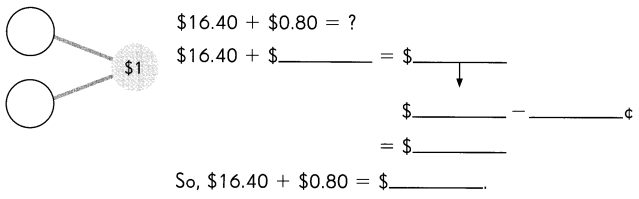Answer: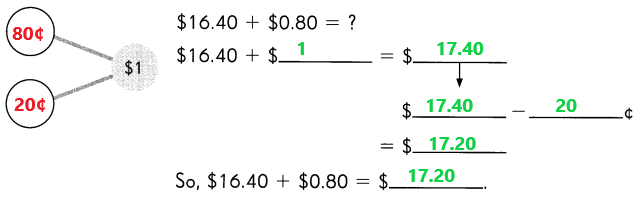Explanation: To make$0.80 to the nearest integer we have to add required ¢ to the $0.80. A number bond is a simple addition of two numbers that add up to give the sum. Add these two numbers 80¢ and 20¢ the sum is$1.
In place of $0.80 we have to use$1. Add $16.40 with$1 the sum is $17.40. The ¢ which are added to the given number$0.80 to make nearest integer are subtracted from the above sum.
Subtract 20¢ from $17.40 the difference is$17.20.
By adding $16.40 with$0.80 the sum is also $17.20. Question 6.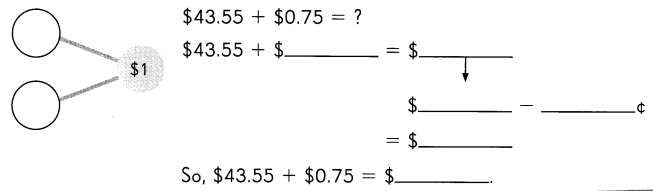Answer: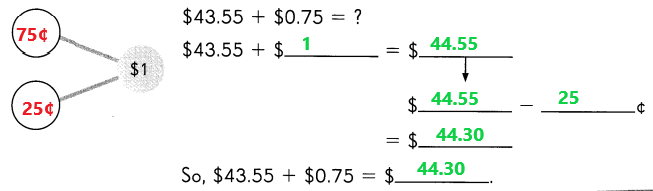Explanation: To make$0.75 to the nearest integer we have to add required ¢ to the $0.75. A number bond is a simple addition of two numbers that add up to give the sum. Add these two numbers 75¢ and 25¢ the sum is$1.
In place of $0.75 we have to use$1. Add $43.55 with$1 the sum is $44.55. The ¢ which are added to the given number$0.75 to make nearest integer are subtracted from the above sum.
Subtract 25¢ from $44.55 the difference is$44.30.
By adding $43.55 with$0.75 the sum is also \$44.30.